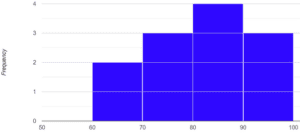# Histograms Worksheets

• ###### Tally Frequency Distribution

Imagine you have some data or information about something you’re interested in, like the number of books people in your class read in a month. A histogram worksheet will give you a set of data and guide you through creating a histogram to represent that data visually. It’s like making a picture that shows how the numbers are spread out.

On these histogram worksheets, you’ll usually see a table or a list of numbers. Each number represents a different value or category, like the number of books read by different students. The worksheet will ask you to group these numbers into intervals or ranges, like 0-5 books, 6-10 books, 11-15 books, and so on. These groups are called “bins.”

Next, you’ll create a vertical or horizontal axis, just like the x and y axes on a graph, but with labels specific to your data. Let’s say you’re making a histogram of the number of books read. The vertical axis might represent the frequency or the number of students who fall into each bin. The horizontal axis will show the different bins or ranges you created earlier.

Now comes the fun part! You’ll take the numbers from the table and count how many fall into each bin. For example, you might count that 3 students read between 0 and 5 books, and 5 students read between 6 and 10 books. You’ll then plot these counts as bars on the histogram. The height of each bar represents the frequency or the number of students in that particular bin.

Once you’ve plotted all the bars, you’ll step back and see the histogram taking shape. It’ll look like a series of connected bars, each representing a range of values and showing how many students fall into that range. The taller the bar, the more students in that range. This way, you can easily see which range has the highest or lowest number of students.

Histogram worksheets also help you interpret the data represented in the histogram. You might be asked questions like, “Which range has the most students?” or “Are there more students who read fewer books or more books?” These questions help you understand the distribution of data and draw conclusions based on the visual representation.

These worksheets are a great way to practice data analysis and visualization skills. They make it easier to understand and present data in a clear and organized manner. Plus, they can be used in various subjects like math, science, or even social studies when you’re studying statistics or analyzing data.

### What Are Histograms?

Histograms are a type of graphical representation used to display the distribution of a continuous dataset by grouping the data into a series of intervals or bins. Each bin represents a range of values, and the height of each bar in the histogram corresponds to the frequency (number of occurrences) or density (percentage) of the data points that fall within that specific bin.

Histograms are particularly useful for understanding the shape and spread of a dataset, such as identifying patterns, trends, or outliers. They can provide insights into the central tendency (mean, median, mode), dispersion (range, variance, standard deviation), and the overall distribution (normal, skewed, bimodal) of the data.

For example, if you have a dataset of test scores for a large group of students, you can create a histogram to visualize the distribution of scores. The x-axis would represent score ranges (bins), and the y-axis would represent the frequency or density of students who achieved scores within each bin. By examining the shape of the histogram, you can gain insights into the overall performance of the students and identify any trends or outliers in the data.

A class of 12 students receive the following scores out a possible 100:

88, 67, 98, 75, 85, 62, 76, 80, 90, 76, 91, 83

We would create a histogram like this:We can quickly understand the frequency distribution of the scores. We can understand that a slight majority of the students scored over 80%.

It’s important to note that the choice of bin size and the number of bins can significantly impact the appearance of a histogram. Smaller bins may reveal more details about the data distribution, while larger bins may provide a more general overview. However, using too few or too many bins can lead to a misleading representation of the data, so it’s crucial to choose an appropriate bin size and number to accurately visualize the dataset.